### IMO Shortlist 2016 problem N1

Kvaliteta:
Avg: 0,0
Težina:
Avg: 6,0

For any positive integer$k$, denote the sum of digits of$k$ in its decimal representation by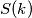$S(k)$. Find all polynomials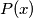$P(x)$ with integer coefficients such that for any positive integer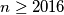$n \geq 2016$, the integer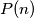$P(n)$ is positive and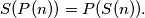Izvor: https://www.imo-official.org/problems/IMO2016SL.pdf